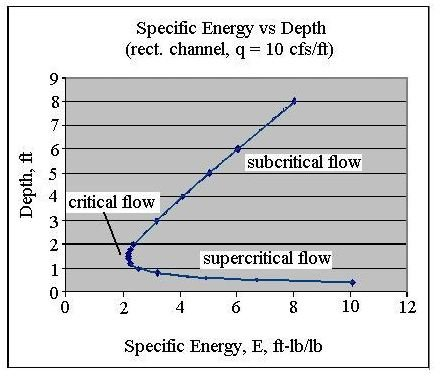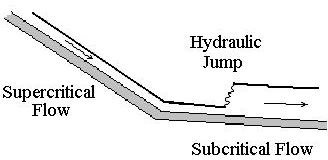# Use the Froude Number, Critical Depth, and Flow Velocity to Find If Open Channel Flow is Subcritical, Critical or Supercritical Flow

## Types of Open Channel Flow

Supercritical flow, subcritical flow, and critical flow are categories of open channel flow. Any open channel flow must be in one of these three categories. Supercritical flow is generally high flow velocity, shallow flow (less than critical depth) on a steep channel slope that has Froude number greater than one. Subcritical flow, on the other hand, is characterized by slower flow velocity, deeper flow depth (more than critical depth) on a shallow channel slope with Froude number less than one. Critical flow is the dividing condition between supercritical flow and subcritical flow.

## Conditions for Critical, Subcritical and Supercritical Flow

Let’s take a look at the conditions for critical flow first. It’s the dividing line between subcritical and supercritical flow. The parameter, specific energy, is often used to introduce the concepts of critical, subcritical, and supercritical flow. The specific energy of a liquid flowing in an open channel is defined to be the sum of its kinetic and potential energy per unit weight of flowing liquid, relative to the channel bottom.

Thus: E = y + V2/2g, whereE = specific energy, ft-lb/lb,

y = depth of flow above the channel bottom, ft,

g = acceleration due to gravity = 32.2 ft/sec2, and

V = average flow velocity, ft/sec, = Q/A, where

Q = volumetric flow rate, cfs, and

A = cross-sectional area of flow normal to the direction of flow, ft2.

The figure at the right above, shows a plot of specific energy versus depth of flow for a particular rectangular channel example. It shows the general pattern for how specific energy, E, varies with depth. At large values of depth, E is large because y is large. For very small values of y, E is large because the flow velocity, V, becomes large, making the kinetic energy large. In between there will be a point at which the specific energy, E, is a minimum. This is the point that is defined to have critical flow conditions (occurring a depth of flow = critical depth). Flows with a shallower depth and higher flow velocity are called supercritical flow and flows with a higher depth and smaller flow velocity are called subcritical flow, as shown on the diagram.

By using a little calculus (setting dE/dy = 0 and solving for y), it can be shown that yc = (q2/g)1/3, for a rectangular channel, where

yc = critical depth (depth of flow at critical flow conditions), ft,

q = Q/b, cfs/ft, and

b = width of the rectangular channel, ft.

## The Froude Number as Related to Critical Flow

The Froude Number is a dimensionless parameter used in connection with open channel flow. For flow in a rectangular channel, the Froude Number is defined as: Fr = V/(gy)1/2, where the variables in the definition are as defined in the previous section. The equation for critical depth in the previous section, yc = (q2/g)1/3, can be rearranged after substituting q = Q/b and Q = VA = Vby, to give the equation: V2/gyc = 1, or in other words, Fr2 = 1, or Fr =1 for critical flow conditions. This can be extended to give the following results:

for Fr < 1, flow is subcritical

for Fr = 1, flow is critical

for Fr > 1, flow is supercritical

This gives a set of criteria for determining whether a given flow is subcritical, critical, or supercritical flow.

## The Hydraulic JumpA physical situation in which both supercritical flow and subcritical flow will be present is the hydraulic jump. Whenever supercritical flow is taking place on a slope that will not sustain the supercritical flow, then a hydraulic jump will be present to make the abrupt transition from supercritical to subcritical flow. This would occur, for example, when the slope of a channel decreases from a steep slope on which supercritical flow occurs to a mild slope that can only sustain subcritical flow. The figure at the right illustrates this situation.

## This post is part of the series: Open Channel Flow Basics

Open channel flow takes place with a free surface open to the atmosphere, so flow is due to gravity, rather than due to pressure as in pipe flow. The Manning equation applies for uniform flow. A hydraulic jump is nonuniform flow. It occurs for supercritical flow on a subcritical channel slope.